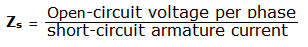Open Circuit Test and Short Circuit Test are performed on a Synchronous Machine to find out the Synchronous impedance For Large Machine to Determine the voltage regulation

Open Circuit Test
the alternator is run at rated synchronous speed and the load terminals are kept open. That is, all the  loads are disconnected. the field current is set to zero,this condition is called open circuit test condition.

The field current is gradually increase in steps, and the terminal voltage Et is measure at each step, The excitation current may be increased  to get 25% more than rated voltage of the alternator. A graph is plotted between the open circuit test voltage Ep and field excitation current If.the characteristic curve so obtain is called open circuit characteristic (O.C.C.). it take the shape of a normal magnetization curve. the extension of linear portion of an O.C.C. is called the air gap line are show in figure.

Short Circuit Test
The armature terminals are shorted through three ammeter.Care should we taken performing this test, and the field current should first decreased to zero before starting the alternator. Each ammeter should have range greater than the full rated value. the alternator run at synchronous speed. then the field current gradually increased in step, and the armature current is measured at each step.

The field current may be increased to get armature current upto 150% of the rated value.The field current If and the average of three ammeter reading at each step is taken.
A graph is plotted between the armature current Ia and field current If. The characteristic so obtained is called short-circuit characteristic (SCC). the characteristic is a straight line as shown in figure.

Calculation of Zs
The open circuit characteristic (O.C.C.) and short circuit characteristic (S.C.C.) are drawn on the same curve sheet. Determine the value of Isc and field current that gives the rated alternator voltage per phase. the synchronous impedance Zs will then be equal to the open circuit voltage divided by the short circuit current at the field current which gives the rated e.m.f. per phase.For the same value of field current.
The synchronous reactance is found as follows.

In figure , consider the field current If = OA that the produces rated alternator voltage per phase. corresponding to this field current the open circuit voltage is AB.

### 20 Comments

1.Great Blog,Thanks for sharing such beautiful information with us. I hope to will share some more information about Short Circuit Analysis. Please visit our website Short Circuit Analysis

2.Its nice but please also show how to find voltage regulation after knowing Xs and Zs.??

3.4.A NICE ANSWER.

5.6.how can I measure field current for brushless excitation? or how does the test changes for brushless excitation? thanks for ur help!

1.What a fucking questions bro!!

7.Can we use. Same Isc..for both o.c.c. and scc test

8.Thank you so much for sharing such an awesome blog...Nice tips! Very well written information. Many thanks!
silent-generators

9.With very simple literature, diagram amd graphical presentation you describe the open and short circuit test of synchronous generator. Thanks a lot.

10.1.generator can convert mechanical energy to electrical energy while alternator can convert both to each other and furthermore a generator that produces alternating current is known as alternator thirdly they also have structural difference

11.12.13.Please write equation

14.How can i find open circuit voltage given the rpms

15.in open circuit test,why the the field curren would be zero when we will open the load??here the path of field current is still closed!!!

16.17.Who invented electrical engineering

18.Heating installation and air conditioning repair. To know more information, visit Ac Repair Dallas